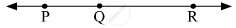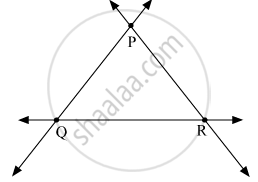Advertisement Remove all ads

# Select the Correct Alternative from the Answer of the Question Given Below. How Many Lines Are Determined by Three Distinct Points? - Geometry

MCQ
Select the correct alternative from the answer of the question given below.
How many lines are determined by three distinct points?

#### Options

• two

• three

• one or three

• six

Advertisement Remove all ads

#### Solution

Consider the 3 distinct points as P, Q and R.
Suppose the points P, Q and R are collinear.So, only one line is determined by the points P, Q and R.
Suppose the points P,Q and R are non collinear.So, three lines can be determined by the points P, Q and R.
Hence, the correct answer is one or three.

Is there an error in this question or solution?
Advertisement Remove all ads

#### APPEARS IN

Balbharati Mathematics 2 Geometry 9th Standard Maharashtra State Board
Chapter 1 Basic Concepts in Geometry
Problem set 1 | Q 1.3 | Page 11
Advertisement Remove all ads
Advertisement Remove all ads
Share
Notifications

View all notifications

Forgot password?